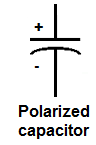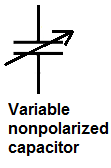﻿# Capacitor Schematic SymbolsSo there are basically 4 main type of capacitor symbols.

There are polarized capacitors, such as electrolytic capacitors.

There are nonpolarized capacitors, such as ceramic capacitors.

And there are variable capacitors, polarized or nonpolarized.

Polarized capacitors are capacitors which have positive and negative terminals. You have to be careful of which side you insert into a part of the circuit. With polarized capacitors, being that you have to observe charge, you can insert it into a circuit the wrong way and the circuit may not work. So you have to observe which terminal goes where.

Nonpolarized capacitors are capacitors which either lead can act as the negative or positive terminal. So it doesn't matter which lead is connected to a certain side of a circuit.

Variable capacitors are capacitors which can change their capacitance values. These might do used in circuits that require adjusting the frequency such as tuning circuits. These can be either polarized or nonpolarized.

So below are the schematic symbols.

Below is the schematic symbol for a polarized capacitor, such as would be the case for an electrolytic capacitor.Below is the schematic symbol for a nonpolarized capacitor, such as would be the case for a ceramic capacitor.Below is the schematic symbol for a variable polarized capacitor.Below is the schematic symbol for a variable nonpolarized capacitor.Related Resources

Electronic Schematic Symbols

Transistor Schematic Symbols

Types of Transistors

Capacitor Equations